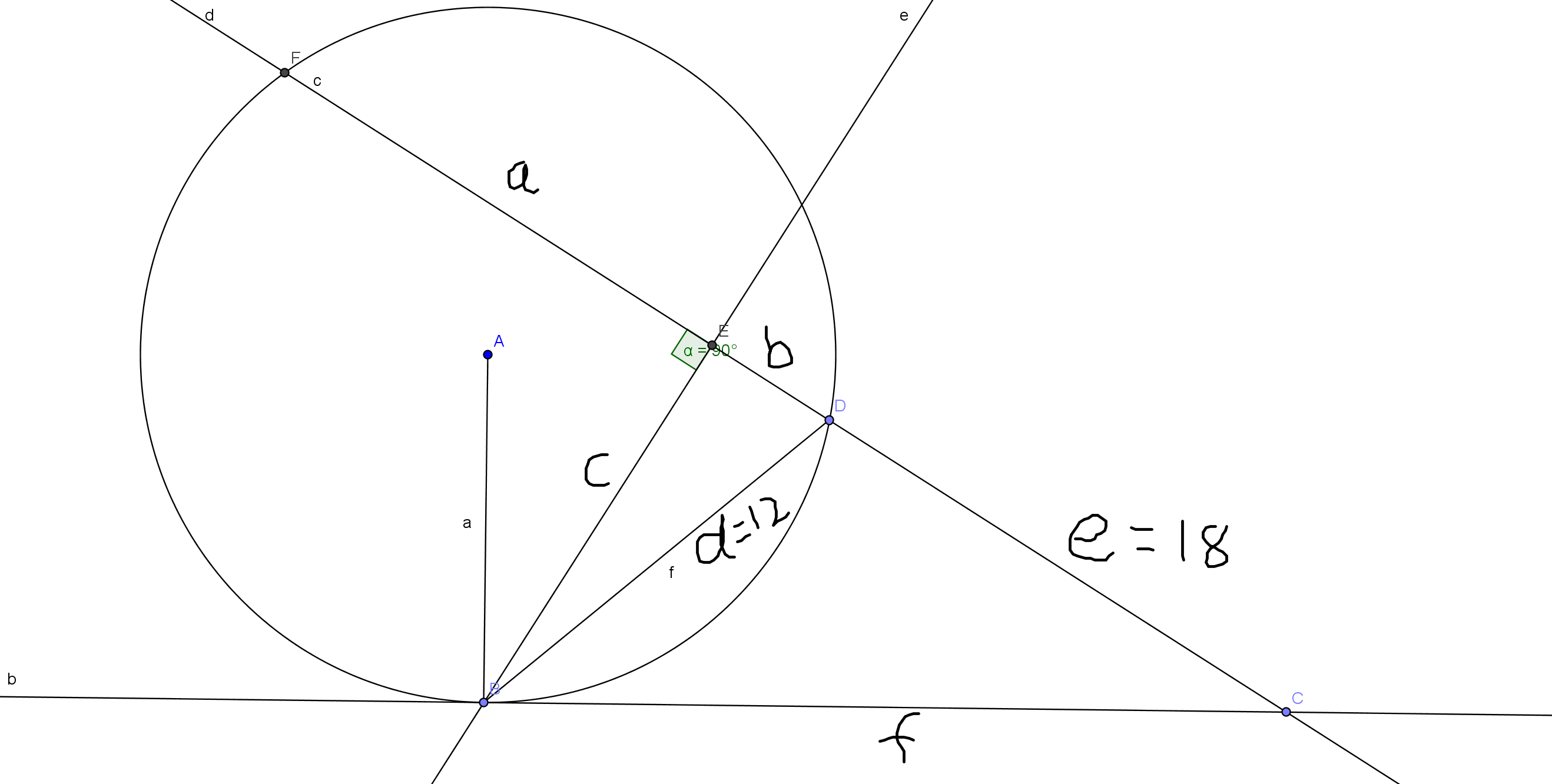# Awesome geometry - 10

Geometry Level 5Consider a circle with center$A$.

Consider point $C$ outside the circle .

Let Point $B$ be on the circle such that $\overline{CB}$ is tangent to the circle.

Consider a line passing through Point $C$ , which intersects the circle at points $D, F$.

Let $\overline{BE}\perp \overline{CF}$

If $\overline{BD}= 12 , \overline{CD}= 18$,

Find $\overline{DF} - 2 \times \overline{DE}$

×